# tensorflow简介--02

1. 我们有房屋面积和房屋价格的数据（图中灰色原点）

2. 用线性回归来建模（红色虚线）

3. 通过最小化损失函数（图中蓝线的长度的平方和）来找出最优的模型的Wb

4. 给定房屋面积，应用建立的线性模型来预测房屋价格（图中蓝色虚线对应的点）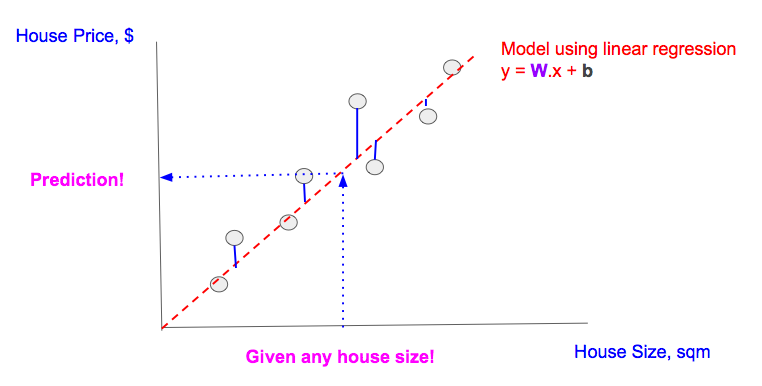## 线性回归模型

Linear Model (in TF notation): y = tf.matmul(x,W) + b


## Training Illustrated

Cost function (in TF notation): tf.reduce_mean(tf.square(y_ - y))


# ... (snip) Variable/Constants declarations (snip) ...
# [A] TF.Graph
y = tf.matmul(x,W) + b
cost = tf.reduce_mean(tf.square(y_-y))
# [B] Train with fixed 'learn_rate'
learn_rate = 0.1
train_step =
for i in range(steps):
# [C] Prepare datapoints
# ... (snip) Code to prepare datapoint as xs, and ys (snip) ...
# [D] Feed Data at each step/epoch into 'train_step'
feed = { x: xs, y_: ys }
sess.run(train_step, feed_dict=feed)


A当中的线性模型和损失函数可以被表示成下面的TF流程图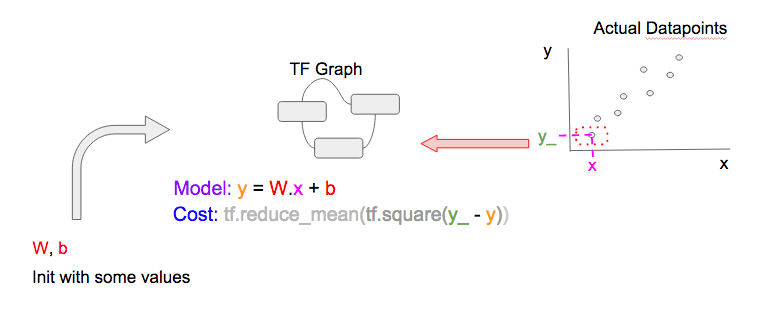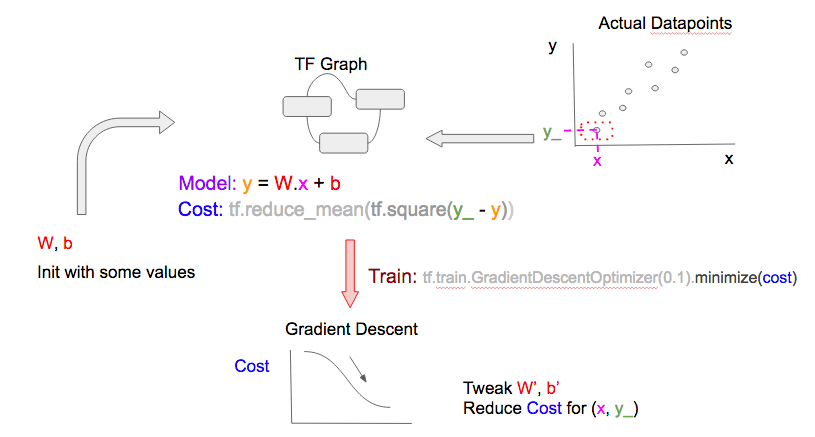• 大部分时候，更多的数据点得到的模型会更好

• 如果你的epochs比你的数据点还多，你可以重复利用数据点，这是没有问题的。梯度下降的优化器总是使用数据点和损失函数(从相应的epoch中的Wb和数据点计算出来)来调整Wb。优化器可能已经用过这个数据点，但是因为损失函数不同，所以它仍然会得到新的信息，也会得到新的调整过的Wb

## Training variation

### stochastic，mini-batch, batch

• 每个epoch中输入到TF.Graph的数据点(右上角的数据点)不同
• 用来调整Wb的梯度下降优化器所用到的数据点（右下角）也不相同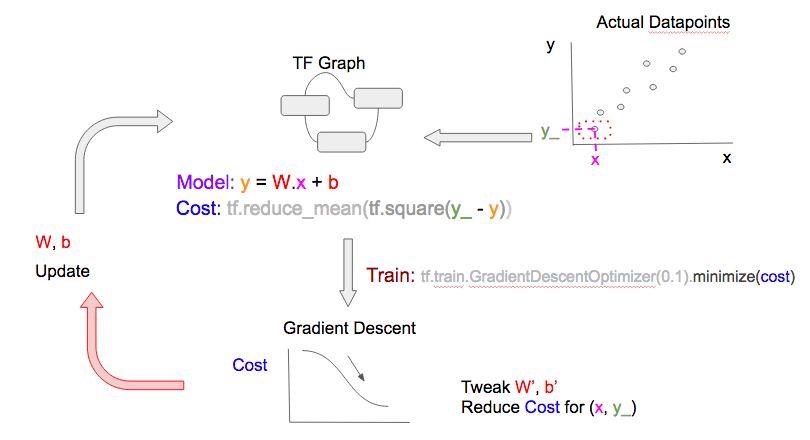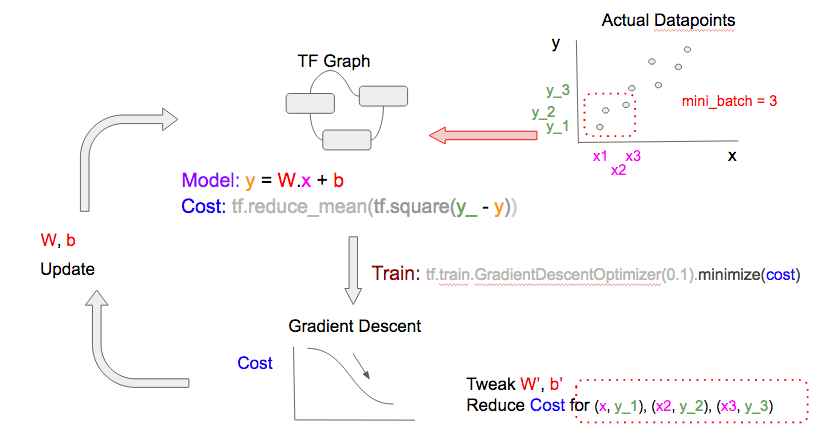• 计算损失函数和梯度下降的计算资源(加减乘)会减少
• 模型学习的速度会提高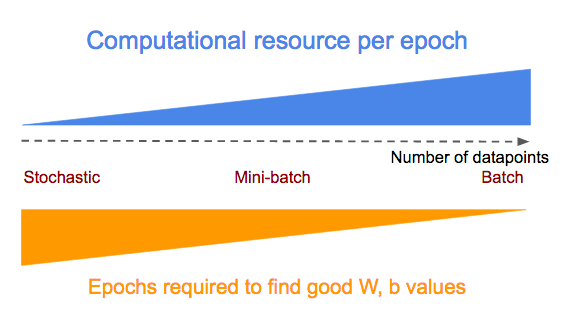# * all_xs: All the feature values
# * all_ys: All the outcome values
# datapoint_size: Number of points/entries in all_xs/all_ys
# batch_size: Configure this to:
#             1: stochastic mode
#             integer < datapoint_size: mini-batch mode
#             datapoint_size: batch mode
# i: Current epoch number
if datapoint_size == batch_size:
# Batch mode so select all points starting from index 0
batch_start_idx = 0
elif datapoint_size < batch_size:
# Not possible
raise ValueError(“datapoint_size: %d, must be greater than
batch_size: %d” % (datapoint_size, batch_size))
else:
# stochastic/mini-batch mode: Select datapoints in batches
#                             from all possible datapoints
batch_start_idx = (i * batch_size) % (datapoint_size — batch_size)
batch_end_idx = batch_start_idx + batch_size
batch_xs = all_xs[batch_start_idx:batch_end_idx]
batch_ys = all_ys[batch_start_idx:batch_end_idx]

# Get batched datapoints into xs, ys, which is fed into
# 'train_step'
xs = np.array(batch_xs)
ys = np.array(batch_ys)


### 使用tf.placeholder建Learn Rate

# Modify [B] to make 'learn_rate' a 'tf.placeholder'
# and supply it to the 'learning_rate' parameter name of
learn_rate = tf.placeholder(tf.float32, shape=[])
learning_rate=learn_rate).minimize(cost)
# Modify [D] to include feed a 'learn_rate' value,
# which is the 'initial_learn_rate' divided by
# 'i' (current epoch number)
# NOTE: Oversimplified. For example only.
feed = { x: xs, y_: ys, learn_rate: initial_learn_rate/i }
sess.run(train_step, feed_dict=feed)


## 总结

### 下一章

• 设置Tensor Board来可视化TF的执行，这样可以检测到我们模型，损失函数和梯度下降的问题
• 展示一个多特征(features)的线性回归模型